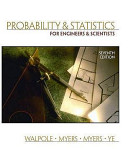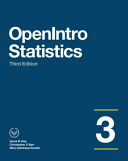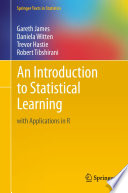### Popis predmeta

#### Course Description

Statistics plays a vital role in every human activity, while the ability to use statistical inferential methods and interpret the results are essential in engineering and science. This course gives a comprehensive introduction to the methods and practices of computer-based statistical data analysis. The course covers and intertwines four integral aspects of statistical analysis: data, statistical methods, mathematical foundations, and interpretation of results. The first part of the course gives an overview of statistical methods, approaches to data description, and data visualization and exploration methods. The second part is devoted to the foundations of statistical inference and covers the selection, application, and adequacy of parametric statistical tests for numeric and categorical data. The third part considers more advanced topics, such as non-parametric statistics, analysis of variance, and correlation analysis. All concepts are illustrated with examples and problem sets on real data in programming languages R and Python.

#### Learning Outcomes

1. Define main notions in the statistical data analysis
2. Explain mathematical backgrounds of main statistical procedures
3. Apply procedure of data preparation and visualization
4. Apply statistical test on real data
5. Analyze the relation between statistical variables by applying regression analysis and correltion analyis
6. Justify the adequacy of statistical inference for given data
7. Interpret the results of statistical data analysis and explain their practical meaning

#### Forms of Teaching

Lectures

Independent assignments

Laboratory

#### Week by Week Schedule

1. Introduction to statistics, basic concepts in statistics, types of data and Stevens' classification of measurement scales.
2. Descriptive statistics, measures of central tendency and dispersion, graphical displays of data, outliers, transformations of data.
3. Introduction to statistical inference, sampling and experimental design, interval estimates.
4. Testing statistical hypotheses, type I and type II errors, test power, test result interpretation.
5. Statistical inference for metric data, testing means, testing paired data, testing variances.
6. Statistical inference for categorical data, goodness of fit test, test of independence, test of homogeneity.
7. Resampling procedures, Jackknife, bootstrap.
8. Midterm exam
9. Introduction to linear regression, estimation of regression coefficients, testing regression coefficients and prediction, Pearson correlation coefficient.
10. Multiple regression, estimation of multiple regression coefficients, confidence intervals and tests for coefficients, quality of model fit.
11. Logistic regression, estimation of logistic regression coefficients, analysis of logistic regression models.
12. Analysis of variance, one-way and two-way ANOVA model.
13. Nonparametric procedures, Wilcoxon signed-rank test, Mann-Whitney-Wilcoxon test, Kruskal-Wallis test, Spearman correlation coefficient.
14. Alternative approaches to data analysis, Bayesian inference, conjugate distributions, difference in Bayesian and frequentist approaches.
15. Final exam

#### Study Programmes

Computing (study)
Free Elective Courses (5. semester)
Electrical Engineering and Information Technology (study)
Free Elective Courses (5. semester)
Audio Technologies and Electroacoustics (profile)
Free Elective Courses (1. semester) (3. semester)
Communication and Space Technologies (profile)
Free Elective Courses (1. semester) (3. semester)
Computational Modelling in Engineering (profile)
Free Elective Courses (1. semester) (3. semester)
Computer Engineering (profile)
Elective Course of the Profile (1. semester) Elective Courses of the Profile (3. semester)
Computer Science (profile)
Free Elective Courses (1. semester) (3. semester)
Control Systems and Robotics (profile)
Free Elective Courses (1. semester) (3. semester)
Data Science (profile)
Core-elective courses (1. semester)
Electrical Power Engineering (profile)
Free Elective Courses (1. semester) (3. semester)
Electric Machines, Drives and Automation (profile)
Free Elective Courses (1. semester) (3. semester)
Electronic and Computer Engineering (profile)
Free Elective Courses (1. semester) (3. semester)
Electronics (profile)
Free Elective Courses (1. semester) (3. semester)
Information and Communication Engineering (profile)
Elective Courses of the Profile (1. semester) Elective Coursesof the Profile (3. semester)
Network Science (profile)
Free Elective Courses (1. semester) (3. semester)
Software Engineering and Information Systems (profile)
Core-elective courses 1 (1. semester)

#### LiteratureRonald Walpole, Raymond Myers, Sharon Myers, Keying Ye (2012.), Probability and Statistics for Engineers and Scientists,Željko Pauše (1993.), Uvod u matematičku statistiku,David Diez, Christopher Barr, Mine Çetinkaya-Rundel (2015.), OpenIntro Statistics,
Mirta Benšić, Nenad Šuvak (2013.), Primijenjena statistika, Sveučilište J. J. ŠtrosmajeraGareth James, Daniela Witten, Trevor Hastie, Robert Tibshirani (2013.), An Introduction to Statistical Learning, Springer Science & Business Media

#### General

ID 183454
Winter semester
5 ECTS
L3 English Level
L1 e-Learning
45 Lectures
12 Laboratory exercises

Excellent
Very Good
Good
Acceptable

#### Learning Outcomes

1. Define main notions in the statistical data analysis
2. Explain mathematical backgrounds of main statistical procedures
3. Apply procedure of data preparation and visualization
4. Apply statistical test on real data
5. Analyze the relation between statistical variables by applying regression analysis and correltion analyis
6. Justify the adequacy of statistical inference for given data
7. Interpret the results of statistical data analysis and explain their practical meaning

#### Forms of Teaching

Lectures

Independent assignments

Laboratory

#### Week by Week Schedule

1. Introduction to statistics, basic concepts in statistics, types of data and Stevens' classification of measurement scales.
2. Descriptive statistics, measures of central tendency and dispersion, graphical displays of data, outliers, transformations of data.
3. Introduction to statistical inference, sampling and experimental design, interval estimates.
4. Testing statistical hypotheses, type I and type II errors, test power, test result interpretation.
5. Statistical inference for metric data, testing means, testing paired data, testing variances.
6. Statistical inference for categorical data, goodness of fit test, test of independence, test of homogeneity.
7. Resampling procedures, Jackknife, bootstrap.
8. Midterm exam
9. Introduction to linear regression, estimation of regression coefficients, testing regression coefficients and prediction, Pearson correlation coefficient.
10. Multiple regression, estimation of multiple regression coefficients, confidence intervals and tests for coefficients, quality of model fit.
11. Logistic regression, estimation of logistic regression coefficients, analysis of logistic regression models.
12. Analysis of variance, one-way and two-way ANOVA model.
13. Nonparametric procedures, Wilcoxon signed-rank test, Mann-Whitney-Wilcoxon test, Kruskal-Wallis test, Spearman correlation coefficient.
14. Alternative approaches to data analysis, Bayesian inference, conjugate distributions, difference in Bayesian and frequentist approaches.
15. Final exam

#### Study Programmes

Computing (study)
Free Elective Courses (5. semester)
Electrical Engineering and Information Technology (study)
Free Elective Courses (5. semester)
Audio Technologies and Electroacoustics (profile)
Free Elective Courses (1. semester) (3. semester)
Communication and Space Technologies (profile)
Free Elective Courses (1. semester) (3. semester)
Computational Modelling in Engineering (profile)
Free Elective Courses (1. semester) (3. semester)
Computer Engineering (profile)
Elective Course of the Profile (1. semester) Elective Courses of the Profile (3. semester)
Computer Science (profile)
Free Elective Courses (1. semester) (3. semester)
Control Systems and Robotics (profile)
Free Elective Courses (1. semester) (3. semester)
Data Science (profile)
Core-elective courses (1. semester)
Electrical Power Engineering (profile)
Free Elective Courses (1. semester) (3. semester)
Electric Machines, Drives and Automation (profile)
Free Elective Courses (1. semester) (3. semester)
Electronic and Computer Engineering (profile)
Free Elective Courses (1. semester) (3. semester)
Electronics (profile)
Free Elective Courses (1. semester) (3. semester)
Information and Communication Engineering (profile)
Elective Courses of the Profile (1. semester) Elective Coursesof the Profile (3. semester)
Network Science (profile)
Free Elective Courses (1. semester) (3. semester)
Software Engineering and Information Systems (profile)
Core-elective courses 1 (1. semester)

#### LiteratureRonald Walpole, Raymond Myers, Sharon Myers, Keying Ye (2012.), Probability and Statistics for Engineers and Scientists,Željko Pauše (1993.), Uvod u matematičku statistiku,David Diez, Christopher Barr, Mine Çetinkaya-Rundel (2015.), OpenIntro Statistics,
Mirta Benšić, Nenad Šuvak (2013.), Primijenjena statistika, Sveučilište J. J. ŠtrosmajeraGareth James, Daniela Witten, Trevor Hastie, Robert Tibshirani (2013.), An Introduction to Statistical Learning, Springer Science & Business Media

#### General

ID 183454
Winter semester
5 ECTS
L3 English Level
L1 e-Learning
45 Lectures
12 Laboratory exercises